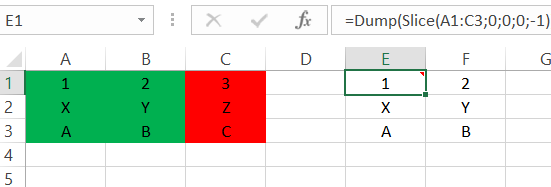``````=Slice(Array, Expr) : array
``````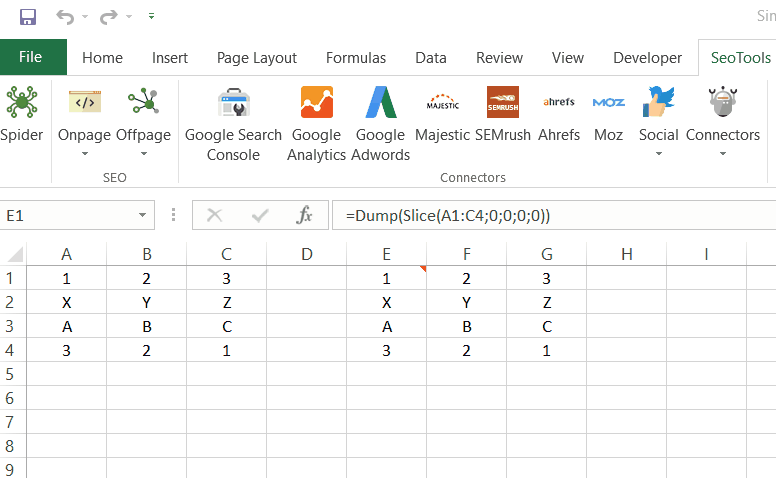## Purpose

Slices an array according to the preferences supplied in the function.

### Array

The two-dimensional field you wish to slice. The array can be expressed with:

1. A Range: `=Slice(A1:B10`

2. Raw inputs: `=Slice({11,12,13;21,22,23;31;32;33`

### Expr

The array can be sliced in four ways:

=Slice(Array,``;``;``;``)

`` Slices the array n steps (rows) from the top: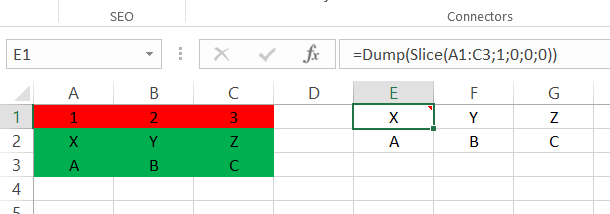`` Slices the array n steps (columns) from the left: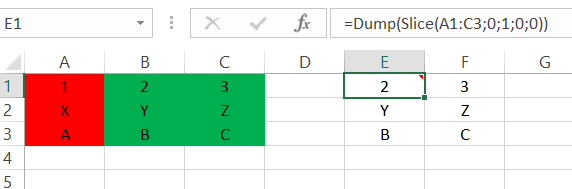`` Slices the array n steps (rows) from the bottom. The input must be a negative value: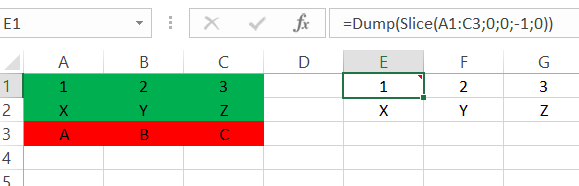`` Slices the array n steps (columns) from the right. The input must be a negative value: